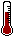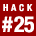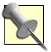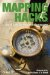# Hack 25. Work with Multiple Lat/Long Formats

### Hack 25 Work with Multiple Lat Long FormatsWhile latitude and longitude stay constant, their representations don't. You can still use new data with a traditional map, if you know a few key conversions.

Even the novice cartographic hacker has noticed that there are at least three common ways to represent latitude and longitude, and at least two conventions for direction. Traditionally, mariners use degree-minute-second (DMS) notation. Computer types find decimal degrees easier to process, and, just to make life more interesting, some people write coordinates with integer degrees and minutes in decimal form. Your GPS receiver may use one style by default, and your mapping tools or your waypoint listing may use another, which can lead to inconvenience and confusion. Fortunately, this situation is pretty easy to deal with.

Latitude and longitude, of course, are just x- and y-coordinates on a great spherical grid that covers the world. Lines of latitude, referred to as parallels (because they never intersect), extend 90 degrees north and south of the equator. Lines of longitude are referred to as meridians and extend 180 degrees west and east of the prime meridian, which passes through the site of the Royal Observatory in Greenwich, England. Southern latitudes and western longitudes are often represented as negative numbers. Minutes are shown with a single quote ('), and seconds are signified with a double quote (").

In traditional notation, one particular spot in the O'Reilly parking lot is at 38 24' 57.636" north latitude, 122 50' 27.348" west longitude.Each minute of change in latitude or longitude at the equator equals one nautical mile, or 6,076 feet. So one second of latitude or longitude is approximately 100 feet. This means that 57.636 seconds of North latitude implies a precision of about an inch, which is well beyond the precision of the GPS used to take this reading.

3.5.1. A Couple of Easy Ways to Convert

If you are working with your own waypoints, you can avoid conversion altogether. Set your GPS to display coordinates in the format that matches your map. On some Garmin units, for example, this is found under Menu [Image_Link]/images/ent/U2192.GIF border=0> Setup [Image_Link]/images/ent/U2192.GIF border=0> Position [Image_Link]/images/ent/U2192.GIF border=0> Position Format.

The easiest way to handle conversions is to let an online coordinate converter do the work. The Federal Communications Commission (FCC) runs one such site at http://www.fcc.gov/mb/audio/bickel/DDDMMSS-decimal.html. You simply enter your coordinates in the format you have, and the coordinates are returned in the format you're after.

3.5.2. Converting Lat/Long to Lat/Long on Paper

But if you want to understand what's actually happening, you need to understand some basic formulas. Going back to the O'Reilly parking lot example, you can convert coordinates from DMS format to decimal-degree format by dividing minutes by 60 and seconds by 3600 and adding all three quantities together to get the desired result:

``` 38
24' 57.636" = 38 + 24/60 + 57.636/3600 = 38.4160

122
50' 27.348" = 122 + 50/60 + 17.348/3600 = -122.8381```

Since this is west of Greenwich, we mark the longitude as negative.

The converse, converting decimal degrees to DMS format, can be accomplished by multiplying the decimal portion of your coordinates by 60; the minutes are the portion of that result to the left of the decimal point, and the seconds can be calculated by taking the remaining fractional part and multiplying it by 60 again. To convert the parking lot's latitude from decimal degrees to DMS:

```38.4160
=
Degrees = 38

Minutes = .4160 * 60 = 24.96'
Seconds = .96 * 60 = 57.60"
= 38
24' 57.60" N```

To get degree/decimal-minute format, just stop when you have the minutes in decimal form (Minutes = .4160 * 60 = 24.96').

3.5.3. Converting Lat/Long to Lat/Long using PROJ.4

PROJ.4 is the open source cartographic projections library. PROJ.4, which can be obtained from http://proj.maptools.org/, includes the Swiss Army Chainsaw of coordinate conversions: a command-line tool called cs2cs. Among the many tricks of cs2cs is its ability to convert lat/long between different formats. The following example converts from decimal degrees to DMS:

```\$ cs2cs +proj=latlong +to +proj=latlong
-122.50 38.75
122d30'W 38d45'N 0.00```

Using the format (-f) parameter, you can change the value from DMS to decimal degrees:

```\$ cs2cs +proj=latlong +to +proj=latlong -f "%.2f"
122d45'W 35d12'N
-122.75 35.20 0.00```

You can also use shell redirection to convert a whole file of coordinates at once:

```\$ cs2cs +proj=latlong +to +proj=latlong < coords.txt
125d19'6.6"E 12d6'29.16"N 0.000
90d33'26.64"W 33d25'56.28"N 0.000
15d30'E 39d18'S 0.000```

See [Hack #26] for other uses of PROJ.4.Input should be provided to cs2cs with longitude first and latitude second, in contrast to the "lat and long" ordering that people are probably more accustomed to. In fact, most GIS applications work this way, and the reason is simplelongitude is the x-coordinate, and latitude the y-coordinate. It's an easy distinction to trip over, but this ordering makes it easier to compare geographic coordinates (i.e., long and lat) to other kinds of coordinate systems.

3.5.4. Converting Lat/Long to Lat/Long in Perl

If you need more fine-grained control over the conversion, you'll probably want to use a program, rather than calculating by hand or using PROJ.4. Walt Mankowski has written the CPAN module Geo::Coordinates::DecimalDegrees, which takes care of the details. Assuming you have a standard Perl installation, you should be able to install it with this command:

`perl -MCPAN -e 'install Geo::Coordinates::DecimalDegrees'`

Here is a bit of Perl to illustrate the use of this module on the same parking lot latitude:

```#!/usr/bin/perl
use Geo::Coordinates::DecimalDegrees;

my (\$deg, \$min, \$sec) = @ARGV;
print "\$deg \$min' \$sec"	DMS
";

my \$decimal_degrees = dms2decimal(\$deg, \$min, \$sec);
print "\$decimal_degrees	Decimal degrees
";

(\$deg, \$min, \$sec) = decimal2dms(\$decimal_degrees);
print "\$deg \$min' \$sec"	Back to DMS
";

(\$deg, \$min) = decimal2dm(\$decimal_degrees);
print "\$deg \$min'	Degrees + decimal minutes
";```

Put this sample code in a file called decimal.pl and execute it to yield the following output:

```\$ perl decimal.pl 38 24 57
38 24' 57" DMS
38.4158333333333 Decimal degrees
38 24' 56.9999999999941" Back to DMS
38 24.9499999999999' Degrees-decimal minutes```

Note that rounding causes some loss of accuracy.Mapping Hacks: Tips & Tools for Electronic Cartography
ISBN: 0596007035
EAN: 2147483647
Year: 2004
Pages: 172